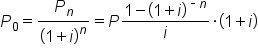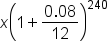+

# Annuities

Author: Sophia Tutorial
##### Description:

Recognize the advantages of annuities and their different types.

(more)### Developing Effective Teams

*No strings attached. This college course is 100% free and is worth 1 semester credit.

37 Sophia partners guarantee credit transfer.

299 Institutions have accepted or given pre-approval for credit transfer.

* The American Council on Education's College Credit Recommendation Service (ACE Credit®) has evaluated and recommended college credit for 32 of Sophia’s online courses. Many different colleges and universities consider ACE CREDIT recommendations in determining the applicability to their course and degree programs.

Tutorial

what's covered
In this lesson, you will learn about the advantages of annuities and their different types. Specifically, this lesson will cover:
1. Annuities
2. Future Value of Annuity
3. Present Value of Annuity
4. Calculating Annuities

## 1. Annuities

An annuity is a type of multi-period investment where there is a certain principal deposited and then regular payments made over the course of the investment. The payments are all a fixed size.

EXAMPLE

A car loan may be an annuity. In order to get the car, you are given a loan to buy the car. In return, you make an initial payment (down payment), and then payments each month of a fixed amount.

There is still an interest rate implicitly charged in the loan. The sum of all the payments will be greater than the loan amount, just as with a regular loan, but the payment schedule is spread out over time.

As a bank, there are three advantages to making the loan an annuity:

1. There is a regular, known cash flow. You know how much money you’ll be getting from the loan and when you’ll be getting them.
2. It should be easier for the person you are loaning to to repay, because they are not expected to pay one large amount at once.
3. It helps them monitor the financial health of the debtor. If the debtor starts missing payments, the bank knows right away that there is a problem, and they could potentially amend the loan to make it better for both parties.
Similar advantages apply to the debtor. There are predictable payments, and paying smaller amounts over multiple periods may be advantageous over paying the whole loan plus interest and fees back at once.

Since annuities, by definition, extend over multiple periods, there are different types of annuities based on when in the period the payments are made:

• Ordinary Annuity: Payments are made at the end of the period. If a period is one month, this means that payments are made on the 28th/30th/31st of each month. Mortgage payments are usually ordinary annuities.
• Annuity-Due: Payments are made at the beginning of the period. For example, if a period is one month, payments are made on the first of each month.
• Perpetuities: Payments continue forever. This is much rarer than the first two types.
terms to know

Annuity
An investment in which regular payments are made over the course of multiple periods.
Ordinary Annuity
An investment with fixed payments that occur at regular intervals, paid at the end of each period.
Annuity-Due
An investment with fixed payments that occur at regular intervals, paid at the beginning of each period.
Perpetuity
An investment in which the periodic payments begin on a fixed date and continue indefinitely.

## 2. Future Value of Annuity

The future value of an annuity is the sum of the future values of all of the payments in the annuity. It is possible to take the FV of all cash flows and add them together, but this isn’t really pragmatic if there are more than a couple of payments. If you were to manually find the FV of all the payments, it would be important to be explicit about when the inception and termination of the annuity is.

For an annuity-due, the payments occur at the beginning of each period, so the first payment is at the inception of the annuity, and the last one occurs one period before the termination.

For an ordinary annuity, however, the payments occur at the end of the period. This means the first payment is one period after the start of the annuity, and the last one occurs right at the end. There are different FV calculations for annuities-due and ordinary annuities because of when the first and last payments occur.

There are some formulas to make calculating the FV of an annuity easier. For both of the formulas we will discuss, you need to know the payment amount (m, though can be written as pmt or p), the interest rate of the account the payments are deposited in (r, though sometimes i), the number of periods per year (n), and the time frame in years (t).

The formula for an ordinary annuity is as follows:

formula

FV of Ordinary AnnuityIn contrast, the formula for an annuity-due is as follows:

formula

FV of Annuity-Duehint
Provided you know m, r, n, and t, therefore, you can find the future value (FV) of an annuity.

## 3. Present Value of Annuity

The Present Value (PV) of an annuity can be found by calculating the PV of each individual payment and then summing them up. As in the case of finding the Future Value (FV) of an annuity, it is important to note when each payment occurs.

Annuities-due have payments at the beginning of each period, and ordinary annuities have them at the end. Recall that the first payment of an annuity-due occurs at the start of the annuity, and the final payment occurs one period before the end. The PV of an annuity-due can be calculated as follows:

formula

PV of Annuity-DueAn ordinary annuity has annuity payments at the end of each period, so the formula is slightly different than for an annuity-due. An ordinary annuity has one full period before the first payment (so it must be discounted) and the last payment occurs at the termination of the annuity (so it must be discounted for one period more than the last period in an annuity-due). The formula is:

formula

PV of Ordinary Annuitybig idea
In both formulas, P is the size of the payment (sometimes A or pmt), i is the interest rate, and n is the number of periods.

Both annuities-due and ordinary annuities have a finite number of payments, so it is possible, though cumbersome, to find the PV for each period. For perpetuities, however, there are an infinite number of periods, so we need a formula to find the PV. The formula for calculating the PV is the size of each payment divided by the interest rate.

## 4. Calculating Annuities

Suppose you have won a lottery that pays \$1,000 per month for the next 20 years. But, you prefer to have the entire amount now. If the interest rate is 8%, how much will you accept?

Consider for argument purposes that two people, Mr. Cash and Mr. Credit, have won the same lottery of \$1,000 per month for the next 20 years. Now, Mr. Credit is happy with his \$1,000 monthly payment, but Mr. Cash wants to have the entire amount now. Our job is to determine how much Mr. Cash should get. We reason as follows: if Mr. Cash accepts x dollars, then the x dollars deposited at 8% for 20 years should yield the same amount as the \$1,000 monthly payments for 20 years. In other words, we are comparing the future values for both Mr. Cash and Mr. Credit, and we would like the future values to be equal.

Since Mr. Cash is receiving a lump sum of x dollars, its future value is given by the lump sum formula:Since Mr. Credit is receiving a sequence of payments, or an annuity, of \$1,000 per month, its future value is given by the annuity formula:The only way Mr. Cash will agree to the amount he receives is if these two future values are equal. So we set them equal and solve for the unknown:So, if Mr. Cash takes his lump sum of \$119,554.29 and invests it at 8% compounded monthly, he will have \$589,020.45 in 20 years.

summary
In this lesson, you learned that annuities are multi-period investments that pay out a regular fixed sum. They can be categorized as ordinary annuities, annuity-due, or perpetuities. The future value of an annuity can be determined by knowing the payment amount, the interest rate where the money is held, the number of annual payments, and the number of years that payments will occur. The present value of an annuity can also be calculated using the same variables. Calculating annuities is useful for making investment choices that maximize returns.

Best of luck in your learning!

Source: THIS CONTENT HAS BEEN ADAPTED FROM LUMEN LEARNING'S “ Annuities” TUTORIAL.

Terms to Know
Annuity

An investment in which regular payments are made over the course of multiple periods.

Annuity-Due

An investment with fixed-payments that occur at regular intervals, paid at the beginning of each period.

Ordinary Annuity

An investment with fixed-payments that occur at regular intervals, paid at the end of each period.

Perpetuity

An investment in which the periodic payments begin on a fixed date and continue indefinitely.

Rating